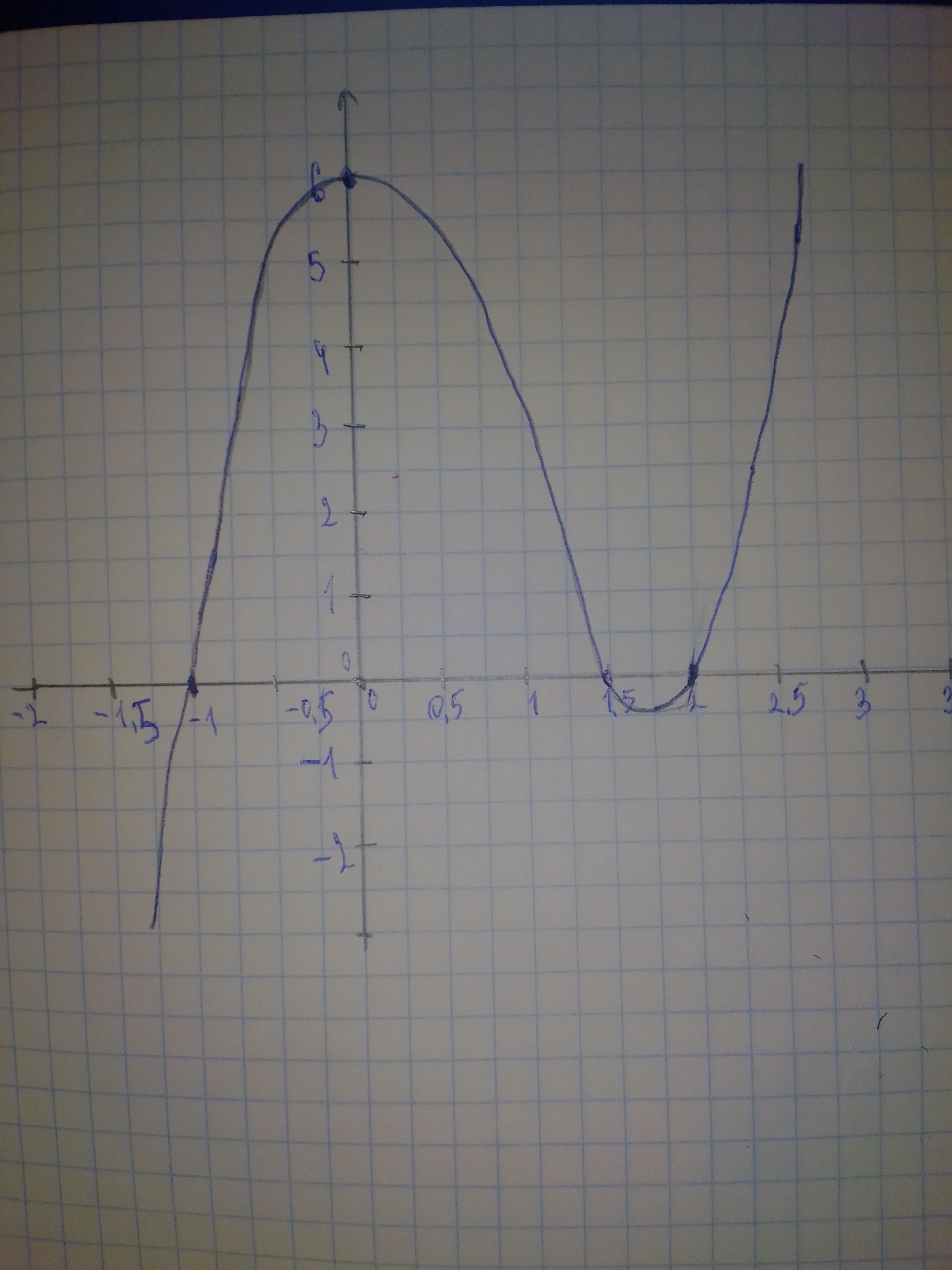Question# Solve the polynomial inequality graphically. 2x^{3}-5x^{2}-x+6>0

Polynomial graphs
ANSWEREDSolve the polynomial inequality graphically. $$\displaystyle{2}{x}^{{{3}}}-{5}{x}^{{{2}}}-{x}+{6}{>}{0}$$Step 1Step 2 $$\displaystyle{2}{x}^{{{3}}}-{5}{x}^{{{2}}}-{x}+{6}{>}{0}$$ To solve graphically we graph $$\displaystyle{f{{\left({x}\right)}}}={2}{x}^{{{3}}}-{5}{x}^{{{2}}}-{x}+{6}$$. A 3rd degree polinomial can have up to 3 zeros, so make sure you can see all the important parts of the graph. We find zeros at: $$\displaystyle{x}=-{1},{1.5},{2}$$ For the inequality, they want $$\displaystyle{f{{\left({x}\right)}}}{>}{0}$$, which are the parts of the graph above the x-axis. We can see from the graph they are the intervals. Answer: $$\displaystyle{\left(-{1},{1.5}\right)}\cup{\left({2},\infty\right)}$$